# Obstruction class

Suppose$X$ is a topological space with a CW-complex structure, and$X^k$ is the$k$-skeleton,$X^{k-1}$ is the$(k-1)$-skeleton. Suppose we have a map from$X^{k-1}$ to$F$. Then, the obstruction class of this fiber bundle is an element of the cellular cohomology group: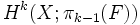$H^k(X; \pi_{k-1}(F))$
For every$k$-cell in$X$, we have an attaching map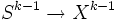$S^{k-1} \to X^{k-1}$. Composing this with the map from$X^{k-1}$ to$F$, we get a map from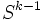$S^{k-1}$ to$F$, yielding an element of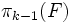$\pi_{k-1}(F)$. Thus, we have a map that associates to every$k$-cell an element of$\pi_{k-1}(F)$. This is precisely an element of the cellular cochain group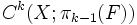$C^k(X; \pi_{k-1}(F))$. The cohomology class of this map is the obstruction class.
This cohomology class can be defined in the more general context of a moving target i.e. the problem of finding a section to a fiber bundle over$X$ with fiber$F$. Here, we require that the fiber bundle is trivial on each of the cells.